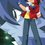# Help me learn my fellow Brilliantians !!!

I am a newbie at Olympiad level mathematics, so I bought some books to help me, but most of the time I find myself stuck at some questions, which frustrates me very much and then leaves me hopeless, I was hoping if you guys might help me. So I'll provide those questions in this discussion, I hope you'll all help me.

1. If $m,n,p,q$ are non negative integers prove that, $\displaystyle \sum_{m=0}^{q} (n-m)\dfrac {(p+m)!}{m!}= \dfrac {(p+q+1)!}{q!} \cdot (\dfrac {n}{p+1}-\dfrac{q}{p+2})$

2. Prove $\dfrac{k^7}{7}+\dfrac{k^5}{5}+\dfrac{2k^3}{3}-\dfrac{k}{105}$ is an positive integer for all positive integers $k$.

3. If $m>n$ prove that $a^{2^ n}+1$ is a divisor of $a^{2^m}-1$. Find LCM of $(a^{2^n}+1, a^{2^m}-1)$.Note by Aejeth Lord
6 years, 9 months ago

This discussion board is a place to discuss our Daily Challenges and the math and science related to those challenges. Explanations are more than just a solution — they should explain the steps and thinking strategies that you used to obtain the solution. Comments should further the discussion of math and science.

When posting on Brilliant:

• Use the emojis to react to an explanation, whether you're congratulating a job well done , or just really confused .
• Ask specific questions about the challenge or the steps in somebody's explanation. Well-posed questions can add a lot to the discussion, but posting "I don't understand!" doesn't help anyone.
• Try to contribute something new to the discussion, whether it is an extension, generalization or other idea related to the challenge.

MarkdownAppears as
*italics* or _italics_ italics
**bold** or __bold__ bold
- bulleted- list
• bulleted
• list
1. numbered2. list
1. numbered
2. list
Note: you must add a full line of space before and after lists for them to show up correctly
paragraph 1paragraph 2

paragraph 1

paragraph 2

[example link](https://brilliant.org)example link
> This is a quote
This is a quote
    # I indented these lines
# 4 spaces, and now they show
# up as a code block.

print "hello world"
# I indented these lines
# 4 spaces, and now they show
# up as a code block.

print "hello world"
MathAppears as
Remember to wrap math in $$ ... $$ or $ ... $ to ensure proper formatting.
2 \times 3 $2 \times 3$
2^{34} $2^{34}$
a_{i-1} $a_{i-1}$
\frac{2}{3} $\frac{2}{3}$
\sqrt{2} $\sqrt{2}$
\sum_{i=1}^3 $\sum_{i=1}^3$
\sin \theta $\sin \theta$
\boxed{123} $\boxed{123}$

Sort by:

I don't really get what your second question means. Do you mean that the expression given will equal to $k$ or other meaning?

- 6 years, 9 months ago

I am really sorry, I made a typo, I have corrected it now.

- 6 years, 9 months ago

If it is interpreted as "find all k such that this polynomial is equal to k when evaluated with k" then we can easily arrive at $k = -1, 0, 1$, and these are unique solutions. But I find that the question is a bit more complicated than this.

- 6 years, 9 months ago

Actually, I believe he is asking to prove that the polynomial is equal to a positive integer for all positive integral $k$, In which case the proof is as follows:

The expression is equivalent to $\frac{1}{105}(15k^7+21k^5+70k^3-k)$, so if we can show that $15k^7+21k^5+70k^3-k$ is divisible by $105=3*5*7$ for all positive integral $k$ then we are done. Consider the expression modulo $3$, $5$, and $7$. We have the equivalences $k^3-k \equiv 0 (mod 3)$, $k^5-k \equiv 0 (mod 5)$, and $k^7-k \equiv 0 (mod 7)$, which are all true for all positive integers $k$ by Fermat's little theorem, so the expression is divisible by $105$.

- 6 years, 9 months ago

Q.3.i have seen this same problem some times ago but with the only difference, there is it mentioned that $a,m,n$ are positive integer so i'm writing my solution assuming $a,m,n$ are positive integer.

we can write,

$(a^{2^{m}}-1)=(a^{2^{m-1}}+1)(a^{2^{m-2}}+1)(a^{2^{m-3}}+1)\dots(a^2+1)(a+1)(a-1)$

let,$n=(m-q)$ where $q\in{{1,2,3,\dots,(m-1)}}$

since,$m>n$ and $n$ is positive

so it is clear that for any value of q, $(a^{2{^n}}+1)$ is a factor of $(a^{2^{m}}-1)$

hence,$(a^{2^{n}}+1)$ is a divisor of $(a^{2^{m}}-1)$ if $m>n$

since, $(a^{2^{n}}+1)$ is a divisor of $(a^{2^{m}}-1)$ so LCM of this two =$(a^{2^{m}}-1)$

- 6 years, 9 months ago

the second question can be done using induction and binomial theroem

- 6 years, 9 months ago

Can you explain.

- 6 years, 9 months ago

let,

$P(k)=\frac{k^{7}}{7}+\frac{k^{5}}{5}+\frac{2k^{3}}{3}-\frac{k}{105}$ is an integer for $k\in+Z$

$P(1)=\frac{1}{7}+\frac{1}{5}+\frac{2}{3}-\frac{1}{105}=1$ which is integer

$P(2)=\frac{2^7}{7}+\frac{2^5}{5}+\frac{2^4}{3}-\frac{2}{105}=30$ this is also integer

let,$P(n)=\frac{n^7}{7}+\frac{n^5}{5}+\frac{2n^3}{3}-\frac{n}{105}=N$ is an integer

where,$n>2$ and $n\in{+Z}$

Therefore $P(n+1)=\frac{(n+1)^7}{7}+\frac{(n+1)^5}{5}+\frac{2(n+1)^3}{3}-\frac{(n+1)}{105}$

$=(\frac{n^7}{7}+\frac{n^5}{5}+\frac{2n^3}{3}-\frac{n}{105})+(\frac{1}{7}+\frac{1}{5}+\frac{2}{3}-\frac{1}{105})+(n^6+3n^5+6n^4+7n^3+7n^2+4n)$

$=P(n)+P(1)+(n^6+3n^5+6n^4+7n^3+7n^2+4n)$

$=N+1+(n^6+3n^5+6n^4+7n^3+7n^2+4n)$

now,$P(1)$ is integer,we assume $P(n)=N$ is integer for some $n\in{+Z}$

and for any value of $n\in{+Z}$, $(n^6+3n^5+6n^4+7n^3+7n^2+4n)$ is integer.

so,$P(n+1)$ is integer.

Thus,when ever the statement is true for $n$ it is true for $(n+1)$.we have checked it is true for k=1,2.so by principle of induction it is true for all positive integer.

- 6 years, 9 months ago

Thank you very much.

- 6 years, 9 months ago